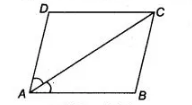# A diagonal of a parallelogram`
Question:

A diagonal of a parallelogram bisects one of its angles. Show that it is a rhombus.

Solution:

Given Let $A B C D$ is a parallelogram and diagonal $A C$ bisects the angle $A$.$\therefore \quad \angle C A B=\angle C A D \quad \ldots$ (i)

To show $A B C D$ is a rhombus.

Proof Since, $A B C D$ is a parallelogram, therefore $A B \| C D$ and $A C$ is a transversal.

$\begin{array}{lll}\therefore & \angle C A B=\angle A C D & \text { [alternate interior angles] }\end{array}$

Again, $A D \| B C$ and $A C$ is a transversal.

$\therefore$  $\angle C A D=\angle A C B$ [alternate interior angles]

So, $\quad \angle A C D=\angle A C B \quad[\because \angle C A B=\angle C A D$, given] $\ldots$ (ii)

Also, $\angle A=\angle C$ [opposite angles of parallelogram are equal]

$\Rightarrow \quad \frac{1}{2} \angle A=\frac{1}{2} \angle C \quad$ [dividing both sides by 2]

$\Rightarrow \quad \angle D A C=\angle D C A \quad$ [from Eqs. (i) and (ii)]

$\Rightarrow \quad C D=A D$

[sides opposite to the equal angles are equal]

But $A B=C D$ and $A D=B C$

[opposite sides of parallelogram are equal]

$\therefore \quad \quad A B=B C=C D=A D$

Thus, all sides are equal. So, $A B C D$ is a rhombus.

Hence proved.